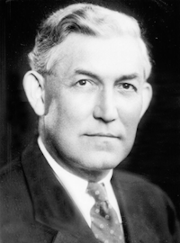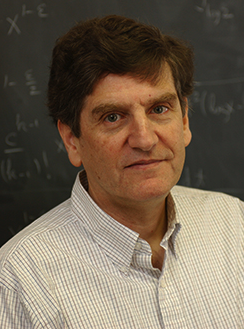Primality Testing and Carmichael Numbers

We know that sometimes Fermat's Little Theorem can provide us evidence that a number is composite. Specifically, whenever an $a^{n-1} \not\equiv 1\pmod{n}$ for some $a$ not divisible by $n$, then we know $n$ is composite. Indeed, when such an $a$ exists, we say $a$ is a Fermat witness to the composite nature of $n$.

We saw something similar with Wilson's Theorem -- namely if $(n-1)! \not\equiv -1\pmod{n}$, we again have evidence that $n$ is composite.

Of course, the converse of Wilson's theorem is also true, which gives us a test for the primality of any given $n$ -- namely, that if and only if $(n-1)! \equiv 1\pmod{n}$, then $n$ is prime. Sadly, of course, this particular primality test is a woefully inefficient one for large $n$ (i.e., values of $n$ with hundreds of digits), given the difficulties associated with calculating $(n-1)!$

Given this, one might naturally wonder: Does the converse of Fermat's Little Theorem hold? Can we somehow build a primality test (perhaps even a more efficient one) from Fermat's Little Theorem?

With regard to the converse of Fermat's Little Theorem -- can we say that if $n$ is composite then for any $a$ not divisible by $n$ it must be the case that $a^{n-1} \not\equiv 1\pmod{n}$?

Sadly, this is not the case. One doesn't even need to look to far for a counter example. Consider the question of whether $n=341$ is prime or composite as suggested by $a=2$:

We wish to determine if $2^{340} \equiv 1\pmod{341}$ or not, so we appeal to fast exponentiation via the method of successive squaring:

$$\begin{array}{rcl} 2^1 & \equiv & 2\\ 2^2 & \equiv & 4\\ 2^4 & \equiv & 16\\ 2^8 & \equiv & 256\\ 2^{16} & \equiv & 256^2 \equiv 64\\ 2^{32} & \equiv & 64^2 \equiv 4 \textrm{ (a repeated value)}\\ 2^{64} & \equiv & 16\\ 2^{128} & \equiv & 256\\ 2^{256} & \equiv & 64\\ \end{array}$$

Then, upon noting which of the above powers are needed to multiply together to get $2^{340}\pmod{341}$, we find:

$$\begin{array}{rcl} 2^{340} & \equiv & 2^{256} \cdot 2^{64} \cdot 2^{16} \cdot 2^4\\ & \equiv & 64 \cdot 16 \cdot 64 \cdot 16\\ & \equiv & 1048576\\ & \equiv & 1 \end{array}$$

So we clearly don't have any evidence that $341$ is composite -- but unfortunately this not enough to force $341$ to be prime. Indeed, the number $341$ is composite, being equal to the product $11 \times 31$.

In such a context, rather than $2$ being a "witness" to the composite nature of $341$, we say $2$ is a Fermat liar with regard to $n$.

I know what you are asking yourself now -- what about the other values of $a$?

We can, of course, for any given $n$, limit our attention to those positive integers $a$ that are less than $n$, since we are working$\pmod{n}$.

We should also note that any $a$ that is not relatively prime to $n$ is automatically a witness to the composite nature of $n$. To see why, suppose this was not the case and such an $a$ was a Fermat liar. Thus, $a^{n-1} \equiv 1\pmod{n}$. Then $1 - a^{n-1} = nk$, or equivalently $a^{n-1} + nk = 1$, for some integer $k$. If $a$ and $n$ share a common divisor $d \gt 1$, we know $d \mid a^{n-1}$ and $d \mid nk$, so $d$ divides their sum, and consequently $d \mid 1$ -- which of course is impossible.

As such, every composite number $n$ will at least have witnesses in the form of multiples of any of its proper divisors (i.e, divisors less than $n$) that are greater than $1$. Still, this is not all that helpful when $n$ is large. Suppose $n=pq$ has $200$ digits with $p$ and $q$, both primes, that each have around $100$ digits. What's the chance of randomly picking an $a$ to test that turns out to be a witness if it must be one a multiple of $p$ or $q$? Given the size of $p$ and $q$, one has to admit that the chances are not very good!

All that said, as we seek to find some witness $a$ to the composite nature of some given $n$, we should use the Euclidean Algorithm to first decide if $gcd(a,n)=1$. If the greatest common divisor is not $1$, we don't have to go any further -- this greatest common factor divides $n$ and we know $n$ is composite with no additional work. Remember, we only look for witnesses in the first place to determine if $n$ is composite. If the greatest common divisor is 1, then we can reduce $a^{n-1}\pmod{n}$ as before (with fast exponentiation) and cross our fingers that it is not congruent to $1$, thus giving us a witness to the composite nature of $n$.

So looking again at $n=341$, what if we looked at $a=3$ instead of $a=2$? A quick check with the Euclidean Algorithm tells us $341$ and $3$ share no common factor besides $1$.

So we proceed to find $3^{340}\pmod{341}$:

Again appealing to fast exponentation,

$$\begin{array}{rcl} 3^1 & \equiv & 3\\ 3^2 & \equiv & 9\\ 3^4 & \equiv & 81\\ 3^8 & \equiv & 81^2 \equiv 82\\ 3^{16} & \equiv & 82^2 \equiv 245\\ 3^{32} & \equiv & 245^2 \equiv 9 \textrm{ (a repeated value)}\\ 3^{64} & \equiv & 81\\ 3^{128} & \equiv & 82\\ 3^{256} & \equiv & 245\\ \end{array}$$

The powers needed to form $3^{340}$ are similar to those needed before:

$$\begin{array}{rcl} 3^{340} & \equiv & 3^{256} \cdot 3^{64} \cdot 3^{16} \cdot 3^4\\ & \equiv & 245 \cdot 81 \cdot 245 \cdot 81\\ & \equiv & 393824025\\ & \equiv & 56 \end{array}$$Robert Daniel Carmichael

Aha! We have discovered that $3$ is a Fermat witness to the composite nature of $341$. One naturally wonders then -- even though some $a$ values for a given number $n$ whose prime or composite nature we hope to determine might be Fermat liars -- how easy are these witnesses to find? We know that any numbers not relatively prime to $n$ must be witnesses -- but these can be hard to find. Just imagine a 100-digit $n$ which is the product of two $50$-digit primes. Randomly picking some value between $2$ and $n$, what are your chances of picking a multiple of one of these two primes? (not very good!) So ignoring these values, must there always be other integers $a$ that for a given composite $n$ can serve as a Fermat witness?

Again, sadly, the answer is no -- although composite values of $n$ with no Fermat witnesses to their composite nature other than those not relatively prime to $n$ are rare. Such values of $n$ are called Carmichael numbers, after Robert Carmichael, an American mathematician from Alabama.

The first few Carmichael numbers are given by 561, 1105, 1729, 2465, 2821, 6601, 8911, 10585, 15841, 29341, 41041, 46657, 52633, 62745, 63973, and 75361. They start to spread out quickly after that, with only 2163 Carmichael numbers below 25,000,000,000. The farther one goes, the rarer these numbers get (e.g., there are only 246,683 below 10,000,000,000,000,000).

As thinly dispersed as they are amongst the larger integers, one might wonder if this is a finite collection of numbers -- or if like the prime numbers, there are an infinite number of them.Carl Bernard Pomerance

While the proof would be too much to get into here, Carl Pomerance (formerly on the faculty of the University of Georgia) in 1992 proved that there are indeed an infinite number of Carmichael numbers.

Even given that there are an infinite number of them -- Carmichael numbers are so spread out that the chance that a randomly chosen number with more than 100 digits is not a Carmichael number is a near certainty. So the chance that in investigating $a^{n-1}\pmod{n}$ for any given very large composite $n$ is $1$ for all $a$ relatively prime to and less than $n$ is remote in the extreme. Still, for such large $n$ checking all of the possible values of $a$ to confirm $a^{n-1} \equiv 1\pmod{n}$ before concluding $n$ is a (probable) prime would be inefficient in the extreme!

Fortunately, there is an interesting relationship between Fermat witnesses and Fermat liars that we can exploit to quickly and efficiently determine if a number is (probably) prime even if it has hundreds of digits!

We've talked already about Carmichael numbers, composite numbers who have no Fermat witnesses. Consider a composite number $n$ with at least one Fermat witness, $b$ and a many distinct Fermat liars, $a_1, a_2, a_3, \ldots, a_m$.

We know that by virtue of being a Fermat witness, $b^{n-1} \not\equiv 1\pmod{n}$.

Also, since each $a_i$ is a Fermat liar, then $a^{n-1} \equiv 1 \pmod{n}$.

Consider $c_i = a_i b$. Note, $$c_i^{n-1} = a_i^{n-1} \cdot b^{n-1} \equiv 1 \cdot b^{n-1} \equiv b^{n-1} \not\equiv 1$$

As such, $c_i$ is a Fermat witness to the composite nature of $n$ as well.

Further, recalling that all of the $a_i$ were distinct, it must be the case that the $c_i$ values are distinct as well. Otherwise, we would have $c_i \equiv c_j\pmod{n}$ for distinct $i$ and $j$, which in turn implies

$$a_i b \equiv a_j b\pmod{n}$$ We have argued before that $b$ must be relatively prime to $n$, so it must also then have an inverse $b^{-1}\pmod{n}$.

Multiplying both sides of the congruence by $b^{-1}$ on the right side, we cancel the $b$'s to reveal $$a_i \equiv a_j\pmod{n}$$ This of course contradicts the distinct nature of the $a_i$ values.

As such, there must be at least as many witnesses $c_i$ as there are liars $a_i$.

Thus, for a given composite $n$, the chances that a randomly selected value less than $n$ that is also relatively prime to $n$ and is a Fermat witness for the composite nature of $n$ is around 50% when there is a Fermat witness to be found.

Consequently, the average number of $a^{n-1}\pmod{n}$ values one must compute before finding a witness (assuming $n$ is not a Carmichael number) is roughly on par or better than the average number of tosses of a coin one must make before seeing "heads" (which is 2, by the way).